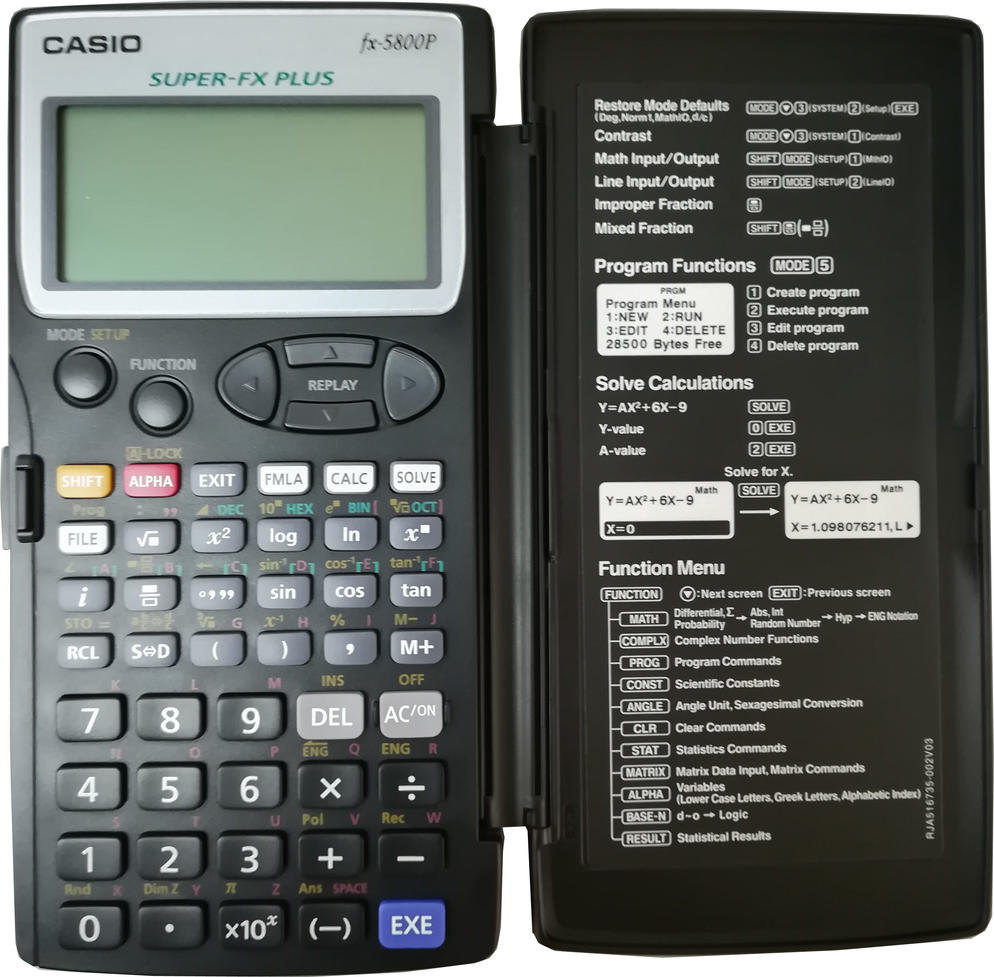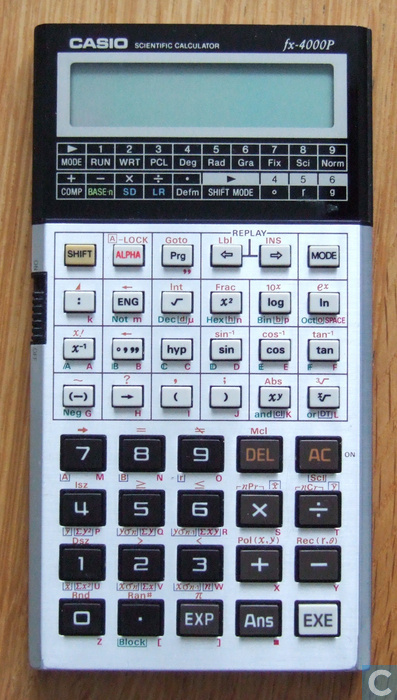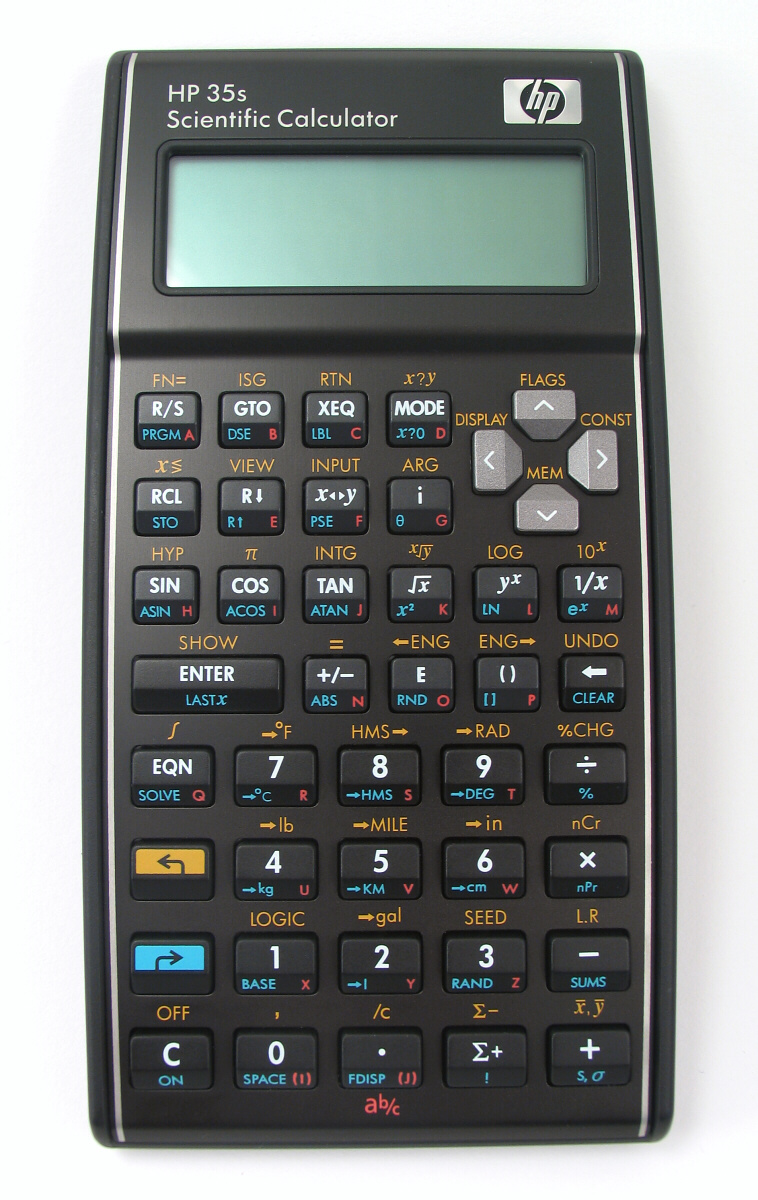Scientific calculators that have formula/equation memory
02-10-2018, 09:51 AM (This post was last modified: 02-10-2018 11:03 AM by martinot.)
Post: #38martinotJunior Member Posts: 40 Joined: Aug 2017
RE: Scientific calculators that have formula/equation memory
(01-05-2018 10:11 AM)pier4r Wrote:  After this thread and the analysis of Dieter (in the same linked thread), I would lean more on the fx5800p rather than the 35s. Moreover the 5800p seems to have persistent memory (with the 35s one has to be careful to swap the batteries, as reported in this thread). This because the 35s and the fx5800p at the moment have prices quite close.

Maybe OT to discuss aesthetics, but even if I think Casio makes some great calculators (and usually with better build quality and functions than TI), why do they to they have to make them so ugly?

Casio used to make great looking calculators!

Look at this "modern" ugly beast from Casio (they almost copied the ugly TI ones):Then look at this sleek and good looking beast:I also like (to be a calculator in production today) the 35s. Much better looking than the current Casios:HP 35s, HP 50g, HP Elite X3, SwissMicros DM42
 « Next Oldest | Next Newest »

 Messages In This Thread Scientific calculators that have formula/equation memory - pier4r - 12-06-2017, 12:18 PM RE: Scientific calculators that have formula/equation memory - Thomas Okken - 12-06-2017, 12:24 PM RE: Scientific calculators that have formula/equation memory - pier4r - 12-06-2017, 12:29 PM RE: Scientific calculators that have formula/equation memory - grsbanks - 12-06-2017, 12:44 PM RE: Scientific calculators that have formula/equation memory - pier4r - 12-06-2017, 01:16 PM RE: Scientific calculators that have formula/equation memory - jebem - 12-06-2017, 01:00 PM RE: Scientific calculators that have formula/equation memory - Gene - 12-06-2017, 01:30 PM RE: Scientific calculators that have formula/equation memory - grsbanks - 12-06-2017, 01:33 PM RE: Scientific calculators that have formula/equation memory - jebem - 12-06-2017, 03:50 PM RE: Scientific calculators that have formula/equation memory - Dave Britten - 12-06-2017, 04:19 PM RE: Scientific calculators that have formula/equation memory - pier4r - 12-06-2017, 09:44 PM RE: Scientific calculators that have formula/equation memory - pier4r - 12-06-2017, 10:22 PM RE: Scientific calculators that have formula/equation memory - jebem - 12-14-2017, 04:04 PM RE: Scientific calculators that have formula/equation memory - Lonewolf - 06-06-2018, 11:53 PM RE: Scientific calculators that have formula/equation memory - ijabbott - 06-07-2018, 01:57 PM RE: Scientific calculators that have formula/equation memory - Dave Britten - 06-07-2018, 02:18 PM RE: Scientific calculators that have formula/equation memory - Lonewolf - 06-07-2018, 07:56 PM RE: Scientific calculators that have formula/equation memory - Dave Britten - 06-07-2018, 08:16 PM RE: Scientific calculators that have formula/equation memory - pier4r - 06-09-2018, 01:38 PM RE: Scientific calculators that have formula/equation memory - Lonewolf - 06-09-2018, 06:16 PM RE: Scientific calculators that have formula/equation memory - pier4r - 12-06-2017, 10:43 PM RE: Scientific calculators that have formula/equation memory - Massimo Gnerucci - 12-07-2017, 12:20 PM RE: Scientific calculators that have formula/equation memory - grsbanks - 12-07-2017, 12:29 PM RE: Scientific calculators that have formula/equation memory - pier4r - 12-07-2017, 12:59 PM RE: Scientific calculators that have formula/equation memory - grsbanks - 12-07-2017, 02:00 PM RE: Scientific calculators that have formula/equation memory - Massimo Gnerucci - 12-07-2017, 08:36 PM RE: Scientific calculators that have formula/equation memory - Chasfield - 12-07-2017, 02:01 PM RE: Scientific calculators that have formula/equation memory - jebem - 12-07-2017, 06:43 PM RE: Scientific calculators that have formula/equation memory - pier4r - 12-07-2017, 07:38 PM RE: Scientific calculators that have formula/equation memory - Csaba Tizedes - 12-09-2017, 11:47 AM RE: Scientific calculators that have formula/equation memory - pier4r - 12-09-2017, 12:06 PM RE: Scientific calculators that have formula/equation memory - Csaba Tizedes - 12-09-2017, 12:29 PM RE: Scientific calculators that have formula/equation memory - pier4r - 12-14-2017, 04:07 PM RE: Scientific calculators that have formula/equation memory - grsbanks - 12-14-2017, 04:23 PM RE: Scientific calculators that have formula/equation memory - Massimo Gnerucci - 12-14-2017, 06:15 PM RE: Scientific calculators that have formula/equation memory - Guenter Schink - 12-14-2017, 10:10 PM RE: Scientific calculators that have formula/equation memory - Massimo Gnerucci - 12-14-2017, 11:01 PM RE: Scientific calculators that have formula/equation memory - klesl - 12-25-2017, 03:22 PM RE: Scientific calculators that have formula/equation memory - pier4r - 12-25-2017, 03:50 PM RE: Scientific calculators that have formula/equation memory - TheKaneB - 01-04-2018, 02:22 AM RE: Scientific calculators that have formula/equation memory - badaze - 01-04-2018, 09:49 PM RE: Scientific calculators that have formula/equation memory - grsbanks - 01-04-2018, 10:10 PM RE: Scientific calculators that have formula/equation memory - badaze - 01-04-2018, 10:24 PM RE: Scientific calculators that have formula/equation memory - pier4r - 01-05-2018, 10:11 AM RE: Scientific calculators that have formula/equation memory - martinot - 02-10-2018 09:51 AM RE: Scientific calculators that have formula/equation memory - JeffJ - 02-16-2018, 06:13 PM RE: Scientific calculators that have formula/equation memory - martinot - 02-16-2018, 09:09 PM RE: Scientific calculators that have formula/equation memory - brickviking - 06-07-2018, 02:18 AM RE: Scientific calculators that have formula/equation memory - Nigel (UK) - 06-07-2018, 04:55 PM

User(s) browsing this thread: 1 Guest(s)